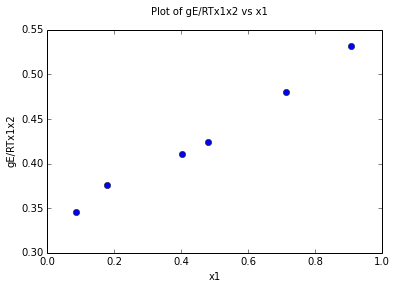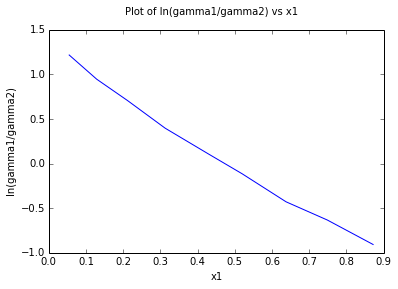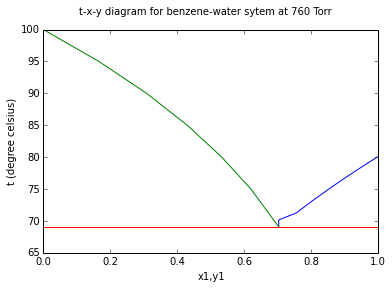# Chapter 12 : Vapor liquid Equilibrium¶

### Example 12.1 Page No : 423¶

In :
%matplotlib inline
import math
from numpy import *
from matplotlib.pyplot import *

# Variables
T = 60.			 #temperature of the system in degree celsius
P = [237.60,265.20,317.50,333.00,368.70,387.20];			 #Pressure data in Torr (from Danneil et al.)
x1 = [0.0870,0.1800,0.4040,0.4790,0.7130,0.9070];			 #mole fraction of benzene in the liquid phase corresponding to the given pressure (no unit) (from Danneil et al.)
y1 = [0.1870,0.3400,0.5780,0.6420,0.7960,0.9220];			 #mole fraction of benzene in the vapour phase corresponding to the given pressure (no unit) (from Danneil et al.)
antoine_const_benzene = [6.87987,1196.760,219.161];			 #Antoine's constants for Benzene from Table A.7
antoine_const_heptane = [6.89386,1264.370,216.640];			 #Antoine's constants for heptane from Table A.7

# Calculations
P1_s = 10**(antoine_const_benzene-(antoine_const_benzene/(T+antoine_const_benzene)));
P2_s = 10**(antoine_const_heptane-(antoine_const_heptane/(T+antoine_const_heptane)));
l = len(P);			 #iteration parameter
i = 0;			 #iteration parameter
gaamma1 = zeros(l)
gaamma2 = zeros(l)
ln_gaamma1_expt = zeros(l)
ln_gaamma2_expt = zeros(l)
gE_RTx1x2 = zeros(l)
while i<l:
gaamma1[i] = (y1[i]*P[i])/(x1[i]*P1_s);
gaamma2[i] = ((1-y1[i])*P[i])/((1-x1[i])*P2_s);
ln_gaamma1_expt[i] = math.log(gaamma1[i]);
ln_gaamma2_expt[i] = math.log(gaamma2[i]);
gE_RTx1x2[i] = ((x1[i]*ln_gaamma1_expt[i])+((1-x1[i])*ln_gaamma2_expt[i]))/(x1[i]*(1-x1[i]));			 # Calculations of gE/RT using Eq.(11.36) (no unit)
i = i+1;

plot(x1,gE_RTx1x2,'o');
suptitle('Plot of gE/RTx1x2 vs x1')
xlabel('x1')
ylabel('gE/RTx1x2');
A21 = 0.555
A12 = 0.315
j = 0
ln_gaamma1 = zeros(l)
ln_gaamma2 = zeros(l)
P_calc = zeros(l)
y1_calc = zeros(l)
gaamma1 = zeros(l)
gaamma2 = zeros(l)
while j<l:
ln_gaamma1[j] = ((1-x1[j])**2)*(A12+(2*(A21-A12)*x1[j]));
ln_gaamma2[j] = (x1[j]**2)*(A21+(2*(A12-A21)*(1-x1[j])));
gaamma1[j] = math.exp(ln_gaamma1[j])
gaamma2[j] = math.exp(ln_gaamma2[j])
P_calc[j] = (gaamma1[j]*x1[j]*P1_s)+(gaamma2[j]*(1-x1[j])*P2_s)
y1_calc[j] = (gaamma1[j]*x1[j]*P1_s)/P[j];
j = j+1;

# Results
print 'Data for the plot of gE/RTx1x2 vs x1: ';

for i in range(l):
print 'P = %f Torr\t x1 = %f\t y1 = %f \t lngamma1) = %f\t\t lngamma2) =\
%f\t\t gE/RTx1x2 = %f'%(P[i],x1[i],y1[i],ln_gaamma1_expt[i],ln_gaamma2_expt[i],gE_RTx1x2[i]);

print 'Results: ';
for i in range(l):
print 'x1 = %f \t gamma1 = %f \t gamma2 = %f \t P_Exptl. = %f \
Torr\t P_Calc = %f Torr\t y1_Exptl = %f \t y1_calc = %f '%(x1[i],gaamma1[i],gaamma2[i],P[i],P_calc[i],y1[i],y1_calc[i]);

Populating the interactive namespace from numpy and matplotlib
Data for the plot of gE/RTx1x2 vs x1:
P = 237.600000 Torr	 x1 = 0.087000	 y1 = 0.187000 	 lngamma1) = 0.265459		 lngamma2) =     0.004741		 gE/RTx1x2 = 0.345243
P = 265.200000 Torr	 x1 = 0.180000	 y1 = 0.340000 	 lngamma1) = 0.246143		 lngamma2) =     0.013576		 gE/RTx1x2 = 0.375599
P = 317.500000 Torr	 x1 = 0.404000	 y1 = 0.578000 	 lngamma1) = 0.148307		 lngamma2) =     0.065399		 gE/RTx1x2 = 0.410716
P = 333.000000 Torr	 x1 = 0.479000	 y1 = 0.642000 	 lngamma1) = 0.130700		 lngamma2) =     0.083082		 gE/RTx1x2 = 0.424313
P = 368.700000 Torr	 x1 = 0.713000	 y1 = 0.796000 	 lngamma1) = 0.049771		 lngamma2) =     0.218778		 gE/RTx1x2 = 0.480259
P = 387.200000 Torr	 x1 = 0.907000	 y1 = 0.922000 	 lngamma1) = 0.005014		 lngamma2) =     0.433207		 gE/RTx1x2 = 0.531539
Results:
x1 = 0.087000 	 gamma1 = 1.346332 	 gamma2 = 1.000884 	 P_Exptl. = 237.600000     Torr	 P_Calc = 238.297793 Torr	 y1_Exptl = 0.187000 	 y1_calc = 0.193066
x1 = 0.180000 	 gamma1 = 1.309835 	 gamma2 = 1.005243 	 P_Exptl. = 265.200000     Torr	 P_Calc = 265.912990 Torr	 y1_Exptl = 0.340000 	 y1_calc = 0.348175
x1 = 0.404000 	 gamma1 = 1.198147 	 gamma2 = 1.044870 	 P_Exptl. = 317.500000     Torr	 P_Calc = 320.705660 Torr	 y1_Exptl = 0.578000 	 y1_calc = 0.597076
x1 = 0.479000 	 gamma1 = 1.159413 	 gamma2 = 1.072467 	 P_Exptl. = 333.000000     Torr	 P_Calc = 335.157916 Torr	 y1_Exptl = 0.642000 	 y1_calc = 0.653147
x1 = 0.713000 	 gamma1 = 1.055628 	 gamma2 = 1.236286 	 P_Exptl. = 368.700000     Torr	 P_Calc = 369.484272 Torr	 y1_Exptl = 0.796000 	 y1_calc = 0.799482
x1 = 0.907000 	 gamma1 = 1.006511 	 gamma2 = 1.521729 	 P_Exptl. = 387.200000     Torr	 P_Calc = 387.326500 Torr	 y1_Exptl = 0.922000 	 y1_calc = 0.923362### Example 12.3 Page No : 430¶

In :
from numpy import *
# Variables
T = 25.     			 #temperature of the system in degree celsius
A12 = 2.0522;			 #three suffix Margules parameters for the system (no unit)
A21 = 1.7201;			 #three suffix Margules parameters for the system (no unit)
P = [118.05,207.70,246.35,259.40,261.50,262.00,261.90,258.70,252.00];			 #Pressure data in Torr (from Tasic et al.)
x1 = [0.0115,0.1125,0.3090,0.5760,0.6920,0.7390,0.7575,0.8605,0.9250];
y1 = [0.1810,0.5670,0.6550,0.7050,0.7250,0.7390,0.7460,0.8030,0.8580];
antoine_const_acetone = [7.11714,1210.595,229.664];			 #Antoine's constants for acetone from Table A.7
antoine_const_chexane = [6.85146,1206.470,223.136];			 #Antoine's constants for cyclohexane from Table A.7

# Calculations
P1_s = 10**(antoine_const_acetone-(antoine_const_acetone/(T+antoine_const_acetone)));
P2_s = 10**(antoine_const_chexane-(antoine_const_chexane/(T+antoine_const_chexane)));

l = len(P)
ln_gaamma1 = zeros(l)
ln_gaamma2 = zeros(l)
gaamma1 = zeros(l)
gaamma2 = zeros(l)
P = zeros(l)
y1_calc = zeros(l)
j = 0;

while j<l:
ln_gaamma1[j] = ((1-x1[j])**2)*(A12+(2*(A21-A12)*x1[j]));
ln_gaamma2[j] = (x1[j]**2)*(A21+(2*(A12-A21)*(1-x1[j])));
gaamma1[j] = math.exp(ln_gaamma1[j]);
gaamma2[j] = math.exp(ln_gaamma2[j]);
P[j] = (gaamma1[j]*x1[j]*P1_s)+(gaamma2[j]*(1-x1[j])*P2_s)
y1_calc[j] = (gaamma1[j]*x1[j]*P1_s)/P[j];
j = j+1;

# Results
print 'P-x-y data: ';
print 'x1 \t  gamma1\t  gamma2 \t P Torr \t y1  ';
for i in range(l):
print '%0.4f \t %f \t %f \t %f  \t %f '%(x1[i],gaamma1[i],gaamma2[i],P[i],y1_calc[i])

P-x-y data:
x1 	  gamma1	  gamma2 	 P Torr 	 y1
0.0115 	 7.372871 	 1.000314 	 116.059350  	 0.168694
0.1125 	 4.747283 	 1.029662 	 212.486878  	 0.580377
0.3090 	 2.415459 	 1.231286 	 255.363338  	 0.674908
0.5760 	 1.350072 	 1.942786 	 259.940648  	 0.690796
0.6920 	 1.163087 	 2.513451 	 261.385489  	 0.711020
0.7390 	 1.112223 	 2.812451 	 261.416681  	 0.726019
0.7575 	 1.095373 	 2.942991 	 261.232545  	 0.733436
0.8605 	 1.029233 	 3.827737 	 256.608420  	 0.796964
0.9250 	 1.008121 	 4.546617 	 248.599211  	 0.866162


### Example 12.4 Page No : 432¶

In :
# Variables
T = 25. 			 #temperature of the system in degree celsius
A = 2.0684;			 #the van Laar parameters for the system (no unit)
B = 1.7174;			 #the van Laar parameters for the system (no unit)
P = [118.05,207.70,246.35,259.40,261.50,262.00,261.90,258.70,252.00];			 #Pressure data in Torr (from Tasic et al.)
x1 = [0.0115,0.1125,0.3090,0.5760,0.6920,0.7390,0.7575,0.8605,0.9250];
y1 = [0.1810,0.5670,0.6550,0.7050,0.7250,0.7390,0.7460,0.8030,0.8580];
antoine_const_acetone = [7.11714,1210.595,229.664];			 #Antoine's constants for acetone from Table A.7
antoine_const_chexane = [6.85146,1206.470,223.136];			 #Antoine's constants for cyclohexane from Table A.7

# Calculations
P1_s = 10**(antoine_const_acetone-(antoine_const_acetone/(T+antoine_const_acetone)))
P2_s = 10**(antoine_const_chexane-(antoine_const_chexane/(T+antoine_const_chexane)));
l = len(P)
j = 0
while j<l:
ln_gaamma1[j] = A/(1+((A*x1[j])/(B*(1-x1[j]))))**2
ln_gaamma2[j] = B/(1+((B*(1-x1[j]))/(A*x1[j])))**2
gaamma1[j] = math.exp(ln_gaamma1[j]);
gaamma2[j] = math.exp(ln_gaamma2[j]);
P[j] = (gaamma1[j]*x1[j]*P1_s)+(gaamma2[j]*(1-x1[j])*P2_s)
y1_calc[j] = (gaamma1[j]*x1[j]*P1_s)/P[j];
j = j+1;

# Results
print 'P-x-y data: ';
i = 0;
print 'x1 \t  gamma1 \t gamma2 \t PTorr \t y1 ';
for i in range(l):
print '%0.4f \t %f \t %f \t %f  \t %f '%(x1[i],gaamma1[i],gaamma2[i],P[i],y1_calc[i]);

P-x-y data:
x1 	  gamma1 	 gamma2 	 PTorr 	 y1
0.0115 	 7.475516 	 1.000328 	 116.333233  	 0.170640
0.1125 	 4.743506 	 1.030586 	 212.468724  	 0.579965
0.3090 	 2.395936 	 1.234218 	 254.168007  	 0.672601
0.5760 	 1.346684 	 1.937830 	 259.284960  	 0.690805
0.6920 	 1.162537 	 2.498302 	 260.842215  	 0.712164
0.7390 	 1.112211 	 2.792268 	 260.900602  	 0.727447
0.7575 	 1.095497 	 2.920797 	 260.729035  	 0.734935
0.8605 	 1.029539 	 3.796506 	 256.244215  	 0.798334
0.9250 	 1.008263 	 4.515793 	 248.404105  	 0.866965


### Example 12.5 Page No : 435¶

In :
import math
from numpy import *
from matplotlib.pyplot import *

# Variables
P = 760.			 #pressure in Torr at which chloroform and methanol form an azeotrope
T = 53.5			 #temperature in degree celsius at which chloroform and methanol form an azeotrope
x1 = 0.65			 #mole fraction of chloroform in the liquid phase (no unit) (corresponding to azeotropic composition)
antoine_const_chloroform = [6.95465,1170.966,226.232];			 #Antoine's constants for acetone from Table A.7
antoine_const_methanol = [8.08097,1582.271,239.726];			 #Antoine's constants for acetone from Table A.7

# Calculations
x2 = 1-x1
P1_s = 10**(antoine_const_chloroform-(antoine_const_chloroform/(T+antoine_const_chloroform)));
P2_s = 10**(antoine_const_methanol-(antoine_const_methanol/(T+antoine_const_methanol)));
gaamma1 = P/P1_s
gaamma2 = P/P2_s
A = math.log(gaamma1)*(1+((x2*math.log(gaamma2))/(x1*math.log(gaamma1))))**2
B = math.log(gaamma2)*(1+((x1*math.log(gaamma1))/(x2*math.log(gaamma2))))**2
x1 = linspace(0.1,0.9,9)
l = len(x1)
gaamma1 = zeros(l)
gaamma2 = zeros(l)
P  = zeros(l)
y1 = zeros(l)
j = 0
while j<l:
ln_gaamma1[j] = A/(1+((A*x1[j])/(B*(1-x1[j]))))**2
ln_gaamma2[j] = B/(1+((B*(1-x1[j]))/(A*x1[j])))**2
gaamma1[j] = math.exp(ln_gaamma1[j]);
gaamma2[j] = math.exp(ln_gaamma2[j]);
P[j] = (gaamma1[j]*x1[j]*P1_s)+(gaamma2[j]*(1-x1[j])*P2_s)
y1[j] = (gaamma1[j]*x1[j]*P1_s)/P[j];
j = j+1;

# Results
print 'VLE data: ';
print 'x1 \tgamma1 \t\t gamma2  \t P Torr \t y1 ';
for i in range(l):
print '%0.1f \t %f \t %f \t %f \t %f '%(x1[i],gaamma1[i],gaamma2[i],P[i],y1[i]);

VLE data:
x1 	gamma1 		 gamma2  	 P Torr 	 y1
0.1 	 2.391069 	 1.005464 	 578.375597 	 0.242663
0.2 	 2.151801 	 1.024547 	 649.357212 	 0.389019
0.3 	 1.928361 	 1.062974 	 699.744047 	 0.485280
0.4 	 1.722251 	 1.130135 	 732.594248 	 0.551969
0.5 	 1.535159 	 1.242383 	 751.239893 	 0.599745
0.6 	 1.369108 	 1.430331 	 759.122391 	 0.635183
0.7 	 1.226766 	 1.756665 	 759.153131 	 0.663976
0.8 	 1.112114 	 2.365222 	 751.206494 	 0.695188
0.9 	 1.031997 	 3.639052 	 721.331744 	 0.755801


### Example 12.6 Page No : 443¶

In :
# Variables
y1 = 0.3;			 #mole fraction of ethane in the vapour phase (no unit)
T = 30. 			 #temperature in degree celsius

# Calculations
y2 = 1-y1
P_guess = 1
K1 = 3.4
K2 = 1.1
x1_calc = y1/K1
x2_calc = y2/K2;
tot = x1_calc+x2_calc
if tot == 1:
P = P_guess
x1 = x1_calc
x2 = x2_calc
else:
P = 1.5
K1 = 2.4
K2 = 0.8
x1 = y1/K1
x2 = y2/K2

# Results
print 'The Dew pressure and the liquid composition of a binary vapour mixture of\
ethane and propane was found to be P = %0.2f MPa\t x1 = %0.3f\t x2 = %0.3f \t'%(P,x1,x2);

The Dew pressure and the liquid composition of a binary vapour mixture of ethane and propane was found to be P = 1.50 MPa	 x1 = 0.125	 x2 = 0.875


### Example 12.7 Page No : 443¶

In :
# Variables
x1 = 0.4;			 #mole fraction of ethane in the liquid phase (no unit)
P = 1.5;			 #pressure in MPa

# Calculations
x2 = 1-x1
t_guess = 10.
K1 = 1.8;
K2 = 0.5;
y1_calc = K1*x1
y2_calc = K2*x2
tot = y1_calc+y2_calc
if tot == 1:
t = t_guess
y1 = y1_calc
y2 = y2_calc
else:
t = 9.
K1 = 1.75
K2 = 0.5;
y1 = K1*x1
y2 = K2*x2

# Results
print 'The bubble temperature and the vapour composition of a binary vapour mixture\
of ethane and propane was found to be t = %d degree celsius y1 = %f\t y2 = %f\t'%(t,y1,y2);

The bubble temperature and the vapour composition of a binary vapour mixture of ethane and propane was found to be t = 9 degree celsius y1 = 0.700000	 y2 = 0.300000


### Example 12.8 Page No : 449¶

In :
from numpy import *
from matplotlib.pyplot import *
%matplotlib inline
# Variables
P = [350.00,446.00,518.00,574.50,609.00,632.50,665.00,681.50,691.50]
x1 = [0.0550,0.1290,0.2120,0.3130,0.4300,0.5200,0.6380,0.7490,0.8720]
y1 = [0.3500,0.5110,0.5990,0.6500,0.6970,0.7260,0.7590,0.8130,0.8830]
antoine_const_propanol = [8.37895,1788.020,227.438];
antoine_const_cbenzene = [7.17294,1549.200,229.260];
T = 95.			 #temperature of the system in degree celsius

# Calculations
P1_s = 10**(antoine_const_propanol-(antoine_const_propanol/(T+antoine_const_propanol)));
P2_s = 10**(antoine_const_cbenzene-(antoine_const_cbenzene/(T+antoine_const_cbenzene)));
l = len(P)
lngamma1_gamma2 = zeros(l)
gaamma1 = zeros(l)
gaamma2 = zeros(l)
i = 0
while i<l:
gaamma1[i] = (y1[i]*P[i])/(x1[i]*P1_s)
gaamma2[i] = ((1-y1[i])*P[i])/((1-x1[i])*P2_s)
lngamma1_gamma2[i] = math.log(gaamma1[i]/gaamma2[i])
i = i+1;

plot(x1,lngamma1_gamma2);
suptitle('Plot of ln(gamma1/gamma2) vs x1')
xlabel('x1')
ylabel('ln(gamma1/gamma2)');
area_above = 1515.
area_below = 1540.
consistency_parameter = abs((area_above-area_below)/(area_above+area_below));

# Results
print 'Values of lngamma1./gamma2: ';
print 'x1 \t gamma1 \t gamma2 \t lngamma1./gamma2';

for i in range(l):
print '%0.4f \t %f \t %f \t %f '%(x1[i],gaamma1[i],gaamma2[i],lngamma1_gamma2[i]);

print 'The value of the consistency parameter = %f'%(consistency_parameter);
if consistency_parameter<0.02 or consistency_parameter == 0.02:
print 'The VLE data is thermodynamically consistent';
else:
print 'The VLE data is not thermodynamically consistent';

Populating the interactive namespace from numpy and matplotlib
Values of lngamma1./gamma2:
x1 	 gamma1 	 gamma2 	 lngamma1./gamma2
0.0550 	 3.266913 	 0.968851 	 1.215489
0.1290 	 2.591375 	 1.007704 	 0.944514
0.2120 	 2.146767 	 1.060854 	 0.704889
0.3130 	 1.749940 	 1.177901 	 0.395847
0.4300 	 1.447924 	 1.302845 	 0.105581
0.5200 	 1.295263 	 1.453040 	 -0.114944
0.6380 	 1.160398 	 1.781713 	 -0.428812
0.7490 	 1.085022 	 2.043343 	 -0.632987
0.8720 	 1.027071 	 2.543757 	 -0.906931
The value of the consistency parameter = 0.008183
The VLE data is thermodynamically consistent

WARNING: pylab import has clobbered these variables: ['f', 'draw_if_interactive', 'new_figure_manager', 'test']
%pylab --no-import-all prevents importing * from pylab and numpy### Example 12.9 Page No : 464¶

In :
from numpy import *
from matplotlib.pyplot import *
%matplotlib inline
# Variables

P = 760.			                            #pressure of the system in Torr
antoine_const_benzene = [6.87987,1196.760,219.161];			 #Antoine's constants for Benzene from Table A.7
t = linspace(60,100,9);			 #temperature range in degree celsius

P2_s = [149.40,187.58,233.71,289.13,355.21,433.51,525.84,634.00,760.00];
x1 = linspace(0,1,6)

# Calculations
l = len(t)
i = 0
P1_s = zeros(l)
P_tot =  zeros(l)

while i<l:
P1_s[i] = 10**(antoine_const_benzene-(antoine_const_benzene/(t[i]+antoine_const_benzene)));
P_tot[i] = P1_s[i]+P2_s[i];
i = i+1;

T = (((t-t)*(760-P_tot))/(P_tot-P_tot))+t

P1_s_three_phase = 10**(antoine_const_benzene-(antoine_const_benzene/(T+antoine_const_benzene)));
P2_s_three_phase = 760-P1_s_three_phase;
y1_three_phase = P1_s_three_phase/760

trange1 = linspace(T,T+11,11)
n = len(trange1)
i = 0
y1 = zeros(n)
P1_s_calc = zeros(n)
while i<n:
if i == 1:
y1[i] = y1_three_phase
else:
P1_s_calc[i] = 10**(antoine_const_benzene-(antoine_const_benzene/(trange1[i]+antoine_const_benzene)));
y1[i] = (P1_s_calc[i])/P
i = i+1;

trange2 = [70,75,80,85,90,95,100]
P2_s_range = [233.71,289.13,355.21,433.51,525.84,634.00,760.00]
p = len(trange2);
i = 0
y_one = zeros(p)
# Calculations of the vapour composition of benzene in the two phase region (L2+V) using Eq.(12.61) (no unit)
y_one[i] = y1_three_phase;
trange2[i] = T;
i = i+1;

while i<p:
y_one[i] = (P-P2_s_range[i])/P;
i = i+1;
k=len(x1)
t_3phase = zeros(k)
i = 0
k = len(x1)
while i<k:
t_3phase[i] = T
i = i+1;

# Results
plot(y1,trange1);
plot(y_one,trange2);
plot(x1,t_3phase);
suptitle('t-x-y diagram for benzene-water sytem at 760 Torr')
xlabel('x1,y1')
ylabel('t (degree celsius)');
q = len(t)
i = 1;
print ' Calculationss performed for determining the three phase equilibrium temperature';
print 'tdegree celsius \t P1_s Torr \t P2_s Torr \t P1_s+P2_s Torr ';
for i in range(q):
print '%d \t \t \t %.2f \t %0.2f \t %.2f '%(t[i],P1_s[i],P2_s[i],P_tot[i]);

print 'The three phase equilibrium temperature = %0.2f degree celsius '%(T);
print 'The vapour phase composition of benzene at the three phase equilibrium point = %0.4f '%(y1_three_phase);

# Note: answer are slightly different because of rounding off error.

Populating the interactive namespace from numpy and matplotlib
Calculationss performed for determining the three phase equilibrium temperature
tdegree celsius 	 P1_s Torr 	 P2_s Torr 	 P1_s+P2_s Torr
60 	 	 	 391.63 	 149.40 	 541.03
65 	 	 	 465.92 	 187.58 	 653.50
70 	 	 	 550.98 	 233.71 	 784.69
75 	 	 	 647.87 	 289.13 	 937.00
80 	 	 	 757.67 	 355.21 	 1112.88
85 	 	 	 881.54 	 433.51 	 1315.05
90 	 	 	 1020.65 	 525.84 	 1546.49
95 	 	 	 1176.21 	 634.00 	 1810.21
100 	 	 	 1349.47 	 760.00 	 2109.47
The three phase equilibrium temperature = 69.06 degree celsius
The vapour phase composition of benzene at the three phase equilibrium point = 0.7028

WARNING: pylab import has clobbered these variables: ['draw_if_interactive']
%pylab --no-import-all prevents importing * from pylab and numpy